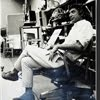## Eugene Ray SDSU 1970'sEugene Ray 1970's. photo credit: Tom Davis

## Monday, June 16, 2014

### BIOTRONIC ARCHITECTURE / gift from our father

+THE BIG PICTURE OF LIFE+
>may take a lifetime to come in focus,
+BIOTRONIC FASCINATION+
^^^^^^^^^^^^^^^^^^
>yes i did earn my first fifty cents at<
>ten years of age stacking lumber<
+(BATON ROUGE 1942+
+BUILDING LESSONS)+
>as the carpenters built houses in<
>our new baton rouge suburb<
^^^^^^^^^^^^^^^^^^^^
+BUT IT WAS OUR FATHERS+
>(he had been in the signal<
>corps & loved electrics)<
+LIGHTED UP MY WORLD+
^^^^^^^^^^^^^^^^^^^^
>this graphic depicting egyptian obelisks as energy<
>sources for ancient alien aerial craft is a favorite,
+(ANCIENT OBELISKS AS+
+ENERGY GENERATORS+
+/ LIGHTS MY OEUVRE)+
>research concept of w.c.bong /david childress<
^^^^^^^^^^^^^^^^^^^^^^^^^^^^
>tesla's vision of aerial craft like the ufo i saw<
>over baton rouge receiving energy from a<
+(THERE IS MUCH RESEARCH+
>series of pylons is very exciting to me<
^^^^^^^^^^^^^^^^^^^^^^^^^^
>the expanding technology of wormhole time &<
>instant space travel makes the old rockets seem<
+(THERE is a SCRAMBLE+
+for INTERDIMENSIONS)+
>like ancient history & bodes well for our future<
^^^^^^^^^^^^^^^^^^^^^^^^^^^^^
>the powerful electro-biological nature of<
>undersea life is an important study for<
+(BIOMORPHIC FUTURE+
+BIO-CITY CONCEPTS)+
>architects of transcendent future cities<
^^^^^^^^^^^^^^^^^^^^^^^
>the "electric love garden" was our first<
>major sdsu architectural exhibition &<
+(BIOTRONIC PROTOTYPES+
+WAS VERY NEW @ SDSU)+
>it attracted eager, enthusiastic crowds<
^^^^^^^^^^^^^^^^^^^^^^^
>our concept of constructing 3 dimensional<
>solid structure via light space holography<
+(NASA NEEDS WAYS TO+
+BUILD VIA ROBOTRY)+
^^^^^^^^^^^^^^^^^^^^^^^^^

>tesla's greatest concept to me is that of<
>energy towers like the ancient obelisk<
+(HIS SPACE CRAFT WAS+
+LIKE MY 1947 CRAFT)+
>groups that energize his space craft<
>(long,silent,silver,cylindrical craft)<
^^^^^^^^^^^^^^^^^^^^^^^^
>my contribution to these biotronic prototypes is<
>a series of transcendental chambers designed to<
+(ART & SCIENCE SYNERGY+
+EARTH'S NEW TREASURE)+
>provide powerful electromagnetic biotronics &<
>a series of transcendent radiant manifestations<
^^^^^^^^^^^^^^^^^^^^^^^^^^^^
>the vision of a radiant architecture first came<
>to me in our glass sansouci forest residence<
+(AT DUSK IT ALL BECAME+
+OUR MAGICAL REALITY)+
>studio when the lights were first turned on<
>(koto music was the perfect sound quality)<
^^^^^^^^^^^^^^^^^^^^^^^^^
>in california i designed a ferro-cement art and<
>architecture university building around open<
+(THE CENTRAL VOLUME+
+BECOMES VERY VITAL)+
>museum exhibition space w/ a plexi-dome<
>(biotronic demonstrations in central space)<
^^^^^^^^^^^^^^^^^^^^^^^^^^^^^
>at san diego state it was my daily pleasure<
>to spread out for the students the books &<
+(1969-1996 WERE YEARS+
+RICH w/ EXPLORATION)+
>photos that opened doors for us all to the<
>questions & answers of our physicality<
^^^^^^^^^^^^^^^^^^^^^^^
>advanced research into orange orbs found<
>in quantity in the night sky reveals work<
+(PARANORMAL RESEARCH+
+BY DAVID M. ROUNTREE)+
>in electromagnetics by secret programs<
^^^^^^^^^^^^^^^^^^^^^^^^^
>(a long way from crystal radios<
>but the latest fascinating work<
>exploraing inter-dimensions)<
+ORBS / (CURRENT BIOTRONICS)+
^^^^^^^^^^^^^^^^^^^^^^^
eugene ray, mfa, architect
professor emeritus, sdsu